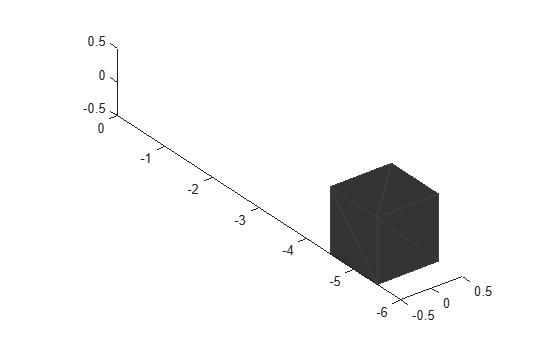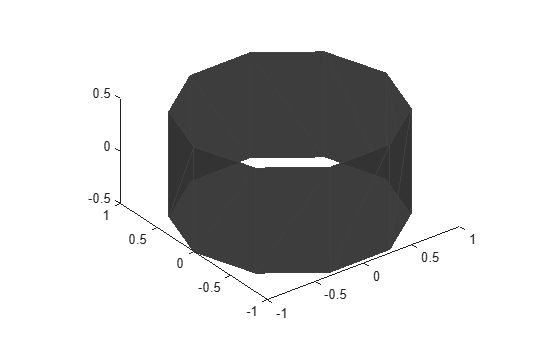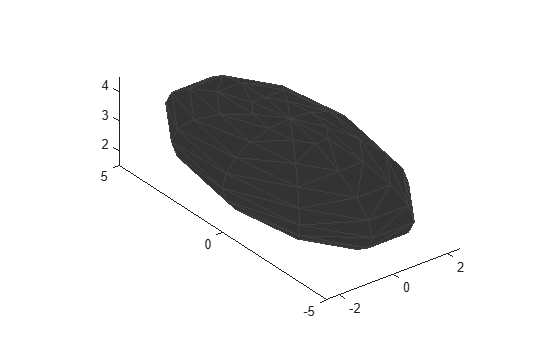# extendedObjectMesh

Mesh representation of extended object

Since R2020a

## Description

The `extendedObjectMesh` represents the 3-D geometry of an object. The 3-D geometry is represented by faces and vertices. Use these object meshes to specify the geometry of an `actor` for simulating lidar sensor data using `lidarPointCloudGenerator`.

## Creation

### Syntax

``mesh = extendedObjectMesh('cuboid')``
``mesh = extendedObjectMesh('cylinder')``
``mesh = extendedObjectMesh('cylinder',n)``
``mesh = extendedObjectMesh('sphere')``
``mesh = extendedObjectMesh('sphere',n)``
``mesh = extendedObjectMesh(vertices,faces)``

### Description

example

````mesh = extendedObjectMesh('cuboid')` returns an `extendedObjectMesh` object, that defines a cuboid with unit dimensions. The origin of the cuboid is located at its geometric center.```

example

````mesh = extendedObjectMesh('cylinder')` returns a hollow cylinder mesh with unit dimensions. The cylinder mesh has 20 equally spaced vertices around its circumference. The origin of the cylinder is located at its geometric center. The height is aligned with the z-axis.```
````mesh = extendedObjectMesh('cylinder',n)` returns a cylinder mesh with `n` equally spaced vertices around its circumference.```

example

````mesh = extendedObjectMesh('sphere')` returns a sphere mesh with unit dimensions. The sphere mesh has 119 vertices and 180 faces. The origin of the sphere is located at its center.```
````mesh = extendedObjectMesh('sphere',n)` additionally allows you to specify the resolution, `n`, of the spherical mesh. The sphere mesh has (`n` + 1)2 - 2 vertices and 2`n`(`n` - 1) faces.```
````mesh = extendedObjectMesh(vertices,faces)` returns a mesh from faces and vertices. `vertices` and `faces` set the `Vertices` and `Faces` properties respectively.```

## Properties

expand all

Vertices of the defined object, specified as an N-by-3 matrix of real scalars. N is the number of vertices. The first, second, and third element of each row represents the x-, y-, and z-position of each vertex, respectively.

Faces of the defined object, specified as a M-by-3 array of positive integers. M is the number of faces. The three elements in each row are the vertex IDs of the three vertices forming the triangle face. The ID of the vertex is its corresponding row number specified in the `Vertices` property.

## Object Functions

Use the object functions to develop new meshes.

 `translate` Translate mesh along coordinate axes `rotate` Rotate mesh about coordinate axes `scale` Scale mesh in each dimension `applyTransform` Apply forward transformation to mesh vertices `join` Join two object meshes `scaleToFit` Auto-scale object mesh to match specified cuboid dimensions `show` Display the mesh as a patch on the current axes

## Examples

collapse all

Create an `extendedObjectMesh` object and translate the object.

Construct a cuboid mesh.

`mesh = extendedObjectMesh('cuboid');`

Translate the mesh by 5 units along the negative y axis.

`mesh = translate(mesh,[0 -5 0]);`

Visualize the mesh.

```ax = show(mesh); ax.YLim = [-6 0];```Create an `extendedObjectMesh` object and visualize the object.

Construct a cylinder mesh.

`mesh = extendedObjectMesh('cylinder');`

Visualize the mesh.

`ax = show(mesh);`Create an `extendedObjectMesh` object and auto-scale the object to the required dimensions.

Construct a sphere mesh of unit dimensions.

`sph = extendedObjectMesh('sphere');`

Auto-scale the mesh to the dimensions in `dims`.

```dims = struct('Length',5,'Width',10,'Height',3,'OriginOffset',[0 0 -3]); sph = scaleToFit(sph,dims);```

Visualize the mesh.

`show(sph);`## Tips

You can use the prebuilt meshes as a starting point to develop your own meshes. The table lists the details of the meshes.

 `driving.scenario.bicycleMesh` Mesh representation of bicycle in driving scenario `driving.scenario.carMesh` Mesh representation of car in driving scenario. `driving.scenario.pedestrianMesh` Mesh representation of pedestrian in driving scenario. `driving.scenario.truckMesh` Mesh representation of truck in driving scenario.

You can view the source files of the meshes to understand how to develop new meshes. At the MATLAB® command line, enter:

`edit driving.scenario.XXXXMesh`
Replace `XXXXMesh` with the name of the mesh.

## Version History

Introduced in R2020a